The REG Procedure

PROC REG Statement

• PROC REG <options>;

The PROC REG statement invokes the REG procedure. The PROC REG statement is required. If you want to fit a model to the data, you must also use a MODEL statement. If you want to use only the PROC REG options, you do not need a MODEL statement, but you must use a VAR statement. If you do not use a MODEL statement, then the COVOUT and OUTEST= options are not available.

Table 97.1 summarizes the options available in the PROC REG statement. Note that any option specified in the PROC REG statement applies to all MODEL statements.

Table 97.1: PROC REG Statement Options

Option

Description

Data Set Options

Names a data set to use for the regression

Outputs a data set that contains parameter estimates and other
model fit summary statistics

Outputs a data set that contains sums of squares and crossproducts

Outputs the covariance matrix for parameter estimates to the
OUTEST= data set

Outputs the number of regressors, the error degrees of freedom,
and the model R square to the OUTEST= data set

Outputs standard errors of the parameter estimates to the
OUTEST= data set

Outputs standardized parameter estimates to the OUTEST= data
set. Use only with the RIDGE= or PCOMIT= option.

Outputs the variance inflation factors to the OUTEST= data set.
Use only with the RIDGE= or PCOMIT= option.

Performs incomplete principal component analysis and outputs
estimates to the OUTEST= data set

Outputs the PRESS statistic to the OUTEST= data set

Performs ridge regression analysis and outputs estimates to the
OUTEST= data set

Same effect as the EDF option

Outputs standard errors, confidence limits, and associated test
statistics of the parameter estimates to the OUTEST= data set

ODS Graphics Options

Produces ODS graphical displays

Display Options

Displays correlation matrix for variables listed in MODEL and
VAR statements

Displays simple statistics for each variable listed in MODEL and
VAR statements

Displays uncorrected sums of squares and crossproducts matrix

Displays all statistics (CORR, SIMPLE, and USSCP)

Suppresses output

Other Options

Sets significance value for confidence and prediction intervals and tests

Sets criterion for checking for singularity

Following are explanations of the options that you can specify in the PROC REG statement (in alphabetical order).

Note that any option specified in the PROC REG statement applies to all MODEL statements.

ALL

requests the display of many tables. Using the ALL option in the PROC REG statement is equivalent to specifying ALL in every MODEL statement. The ALL option also implies the CORR , SIMPLE , and USSCP options.

ALPHA=number

sets the significance level used for the construction of confidence intervals. The value must be between 0 and 1; the default value of 0.05 results in 95% intervals. This option affects the PROC REG option TABLEOUT; the MODEL options CLB, CLI, and CLM; the OUTPUT statement keywords LCL, LCLM, UCL, and UCLM; the PLOT statement keywords LCL., LCLM., UCL., and UCLM.; and the PLOT statement options CONF and PRED.

CORR

displays the correlation matrix for all variables listed in the MODEL or VAR statement.

COVOUT

outputs the covariance matrices for the parameter estimates to the OUTEST= data set. This option is valid only if the OUTEST= option is also specified. See the section OUTEST= Data Set.

DATA=SAS-data-set

names the SAS data set to be used by PROC REG. The data set can be an ordinary SAS data set or a TYPE=CORR, TYPE=COV, or TYPE=SSCP data set. If one of these special TYPE= data sets is used, the OUTPUT , PAINT , PLOT , and REWEIGHT statements, ODS Graphics, and some options in the MODEL and PRINT statements are not available. See Appendix A: Special SAS Data Sets, for more information about TYPE= data sets. If the DATA= option is not specified, PROC REG uses the most recently created SAS data set.

EDF

outputs the number of regressors in the model excluding and including the intercept, the error degrees of freedom, and the model R square to the OUTEST= data set.

NOPRINT

suppresses the normal display of results. Note that this option temporarily disables the Output Delivery System (ODS); see Chapter 20: Using the Output Delivery System, for more information.

OUTEST=SAS-data-set

requests that parameter estimates and optional model fit summary statistics be output to this data set. See the section OUTEST= Data Set for details. If you want to create a SAS data set in a permanent library, you must specify a two-level name. For more information about permanent libraries and SAS data sets, see SAS Language Reference: Concepts.

OUTSEB

outputs the standard errors of the parameter estimates to the OUTEST= data set. The value SEB for the variable _TYPE_ identifies the standard errors. If the RIDGE= or PCOMIT= option is specified, additional observations are included and identified by the values RIDGESEB and IPCSEB, respectively, for the variable _TYPE_. The standard errors for ridge regression estimates and IPC estimates are limited in their usefulness because these estimates are biased. This option is available for all model selection methods except RSQUARE, ADJRSQ, and CP.

OUTSSCP=SAS-data-set

requests that the sums of squares and crossproducts matrix be output to this TYPE=SSCP data set. See the section OUTSSCP= Data Sets for details. If you want to create a SAS data set in a permanent library, you must specify a two-level name. For more information about permanent libraries and SAS data sets, see SAS Language Reference: Concepts.

OUTSTB

outputs the standardized parameter estimates as well as the usual estimates to the OUTEST= data set when the RIDGE= or PCOMIT= option is specified. The values RIDGESTB and ICPSTB for the variable _TYPE_ identify ridge regression estimates and IPC estimates, respectively.

OUTVIF

outputs the variance inflation factors (VIF) to the OUTEST= data set when the RIDGE= or PCOMIT= option is specified. The factors are the diagonal elements of the inverse of the correlation matrix of regressors as adjusted by ridge regression or IPC analysis. These observations are identified in the output data set by the values RIDGEVIF and IPCVIF for the variable _TYPE_.

PCOMIT=list

requests an incomplete principal component (IPC) analysis for each value m in the list. The procedure computes parameter estimates by using all but the last m principal components. Each value of m produces a set of IPC estimates, which are output to the OUTEST= data set. The values of m are saved by the variable _PCOMIT_, and the value of the variable _TYPE_ is set to IPC to identify the estimates. Only nonnegative integers can be specified with the PCOMIT= option.

If you specify the PCOMIT= option, RESTRICT statements are ignored.

PLOTS <(global-plot-options)> <= plot-request<(options)>>
PLOTS <(global-plot-options)> <= (plot-request<(options)> <... plot-request<(options)>>)>

controls the plots produced through ODS Graphics. When you specify only one plot request, you can omit the parentheses around the plot request. Here are some examples:

  plots        = none
plots        = diagnostics(unpack)
plots        = (all fit(stats=none))
plots(label) = (rstudentbyleverage cooksd)
plots(only)  = (diagnostics(stats=all) fit(nocli stats=(aic sbc)))


ODS Graphics must be enabled before plots can be requested. For example:

ods graphics on;

proc reg;
model y = x1-x10;
run;

proc reg plots=diagnostics(stats=(default aic sbc));
model y = x1-x10;
run;

ods graphics off;


For more information about enabling and disabling ODS Graphics, see the section Enabling and Disabling ODS Graphics in Chapter 21: Statistical Graphics Using ODS.

If ODS Graphics is enabled but you do not specify the PLOTS= option, then PROC REG produces a default set of plots. Table 97.2 lists the default set of plots produced.

Table 97.2: Default Graphs Produced

Plot

Conditional On

DiagnosticsPanel

Unconditional

ResidualPlot

Unconditional

FitPlot

Model with one regressor (excluding intercept)

PartialPlot

PARTIAL option specified in MODEL statement

RidgePanel

RIDGE= option specified in PROC REG or MODEL statement

For models with multiple dependent variables, separate plots are produced for each dependent variable. For jobs with more than one MODEL statement, plots are produced for each model statement.

The global-options apply to all plots generated by the REG procedure, unless it is altered by a specific-plot-option. The following global-plot-options are available:

LABEL

specifies that the LABEL option be applied to each plot that supports a LABEL option. See the descriptions of the specific plots for details.

MAXPOINTS=NONE | max <heat-max>

suppresses most plots that require processing more than max points. When the number of points exceeds max but does not exceed heat-max divided by the number of independent variables, heat maps are displayed instead of scatter plots for the fit and residual plots. All other plots are suppressed when the number of points exceeds max. The default is MAXPOINTS=5000 150000. These cutoffs are ignored if you specify MAXPOINTS=NONE.

MODELLABEL

requests that the model label be displayed in the upper-left corner of all plots. This option is useful when you use more than one MODEL statement.

ONLY

suppress the default plots. Only plots specifically requested are displayed.

STATS=ALL | DEFAULT | NONE | (plot-statistics)

requests statistics that are included on the fit plot and diagnostics panel. Table 97.3 lists the statistics that you can request. STATS=ALL requests all these statistics; STATS=NONE suppresses them.

Table 97.3: Statistics Available on Plots

Keyword

Default

Description

x

AIC

Akaike’s information criterion

BIC

Sawa’s Bayesian information criterion

CP

Mallows’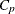statistic

COEFFVAR

coefficient of variation

DEPMEAN

mean of dependent

DEFAULT

all default statistics

EDF

x

error degrees of freedom

GMSEP

estimated MSE of prediction, assuming multivariate normality

JP

final prediction error

MSE

x

mean squared error

NOBS

x

number of observations used

NPARM

x

number of parameters in the model (including the intercept)

PC

Amemiya’s prediction criterion

RSQUARE

x

R-square

SBC

SBC statistic

SP

SP statistic

SSE

error sum of squares

You request statistics in addition to the default set by including the keyword DEFAULT in the plot-statistics list.

UNPACK

suppresses paneling.

USEALL

specifies that predicted values at data points with missing dependent variable(s) be included on appropriate plots. By default, only points used in constructing the SSCP matrix appear on plots.

The following specific plots are available:

displays the adjusted R-square values for the models examined when you request variable selection with the SELECTION= option in the MODEL statement.

The following adjrsq-options are available for models where you request the RSQUARE, ADJRSQ, or CP selection method:

LABEL

requests that the model number corresponding to the one displayed in the "Subset Selection Summary" table be used to label the model with the largest adjusted R-square statistic at each value of the number of parameters.

LABELVARS

requests that the list (excluding the intercept) of the regressors in the relevant model be used to label the model with the largest adjusted R-square statistic at each value of the number of parameters.

AIC <(aic-options)>

displays Akaike’s information criterion (AIC) for the models examined when you request variable selection with the SELECTION= option in the MODEL statement.

The following aic-options are available for models where you request the RSQUARE, ADJRSQ, or CP selection method:

LABEL

requests that the model number corresponding to the one displayed in the "Subset Selection Summary" table be used to label the model with the smallest AIC statistic at each value of the number of parameters.

LABELVARS

requests that the list (excluding the intercept) of the regressors in the relevant model be used to label the model with the smallest AIC statistic at each value of the number of parameters.

ALL

produces all appropriate plots.

BIC <(bic-options)>

displays Sawa’s Bayesian information criterion (BIC) for the models examined when you request variable selection with the SELECTION= option in the MODEL statement.

The following bic-options are available for models where you request the RSQUARE, ADJRSQ, or CP selection method:

LABEL

requests that the model number corresponding to the one displayed in the "Subset Selection Summary" table be used to label the model with the smallest BIC statistic at each value of the number of parameters.

LABELVARS

requests that the list (excluding the intercept) of the regressors in the relevant model be used to label the model with the smallest BIC statistic at each value of the number of parameters.

COOKSD <(LABEL)>

plots Cook’s D statistic by observation number. Observations whose Cook’s D statistic lies above the horizontal reference line at value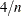, where n is the number of observations used, are deemed to be influential (Rawlings, Pantula, and Dickey 1998). If you specify the LABEL option, then points deemed as influential are labeled. If you do not specify an ID variable, the observation number within the current BY group is used as the label. If you specify one or more ID variables in one or more ID statements, then the first ID variable you specify is used for the labeling.

CP <(cp-options)>

displays Mallows’statistic for the models examined when you request variable selection with the SELECTION= option in the MODEL statement. For models where you request the RSQUARE, ADJRSQ, or CP selection, reference lines corresponding to the equations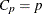and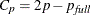, where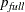is the number of parameters in the full model (excluding the intercept) and p is the number of parameters in the subset model (including the intercept), are displayed on the plot ofversus p. For the purpose of parameter estimation, Hocking (1976) suggests selecting a model where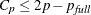. For the purpose of prediction, Hocking suggests the criterion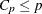. Mallows (1973) suggests that all subset models withsmall and near p  be considered for further study.

The following cp-options are available for models where you request the RSQUARE, ADJRSQ, or CP selection method:

LABEL

requests that the model number corresponding to the one displayed in the "Subset Selection Summary" table be used to label the model with the smalleststatistic at each value of the number of parameters.

LABELVARS

requests that the list (excluding the intercept) of the regressors in the relevant model be used to label the model with the smalleststatistic at each value of the number of parameters.

CRITERIA | CRITERIONPANEL <(criteria-options)>

produces a panel of fit criteria for the models examined when you request variable selection with the SELECTION= option in the MODEL statement. The fit criteria displayed are R-square, adjusted R-square, Mallows’, Akaike’s information criterion (AIC), Sawa’s Bayesian information criterion (BIC), and Schwarz’s Bayesian information criterion (SBC). For SELECTION=RSQUARE, SELECTION=ADJRSQ, or SELECTION=CP, scatter plots of these statistics versus the number of parameters (including the intercept) are displayed. For other selection methods, line plots of these statistics as function of the selection step number are displayed.

The following criteria-options are available:

LABEL

requests that the model number corresponding to the one displayed in the "Subset Selection Summary" table be used to label the best model at each value of the number of parameters. This option applies only to the RSQUARE, ADJRSQ, and CP selection methods.

LABELVARS

requests that the list (excluding the intercept) of the regressors in the relevant model be used to label the best model at each value of the number of parameters. Since these labels are typically long, LABELVARS is supported only when the panel is unpacked. This option applies only to the RSQUARE, ADJRSQ, and CP selection methods.

UNPACK

suppresses paneling. Separate plots are produced for each of the six fit statistics. For models where you request the RSQUARE, ADJRSQ, or CP selection, two reference lines corresponding to the equationsand, whereis the number of parameters in the full model (excluding the intercept) and p is the number of parameters in the subset model (including the intercept), are displayed on the plot ofversus p. For the purpose of parameter estimation, Hocking (1976) suggests selecting a model where. For the purpose of prediction, Hocking suggests the criterion. Mallows (1973) suggests that all subset models withsmall and near p be considered for further study.

DFBETAS <(DFBETAS-options)>

produces panels of DFBETAS by observation number for the regressors in the model. Note that each panel contains at most six plots, and multiple panels are used in the case where there are more than six regressors (including the intercept) in the model. Observations whose DFBETAS’ statistics for a regressor are greater in magnitude than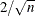, where n is the number of observations used, are deemed to be influential for that regressor (Rawlings, Pantula, and Dickey 1998).

The following DFBETAS-options are available:

COMMONAXES

specifies that the same DFBETAS axis be used in all panels when multiple panels are needed. By default, the DFBETAS axis is chosen independently for each panel. If you also specify the UNPACK option, then the same DFBETAS axis is used for each regressor.

LABEL

specifies that observations whose magnitude are greater thanbe labeled. If you do not specify an ID variable, the observation number within the current BY group is used as the label. If you specify one or more ID variables on one or more ID statements, then the first ID variable you specify is used for the labeling.

UNPACK

suppresses paneling. The DFBETAS statistics for each regressor are displayed on separate plots.

DFFITS <(LABEL)>

plots the DFFITS statistic by observation number. Observations whose DFFITS’ statistic is greater in magnitude than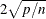, where n is the number of observations used and p is the number of regressors, are deemed to be influential (Rawlings, Pantula, and Dickey 1998). If you specify the LABEL option, then these influential observations are labeled. If you do not specify an ID variable, the observation number within the current BY group is used as the label. If you specify one or more ID variables in one or more ID statements, then the first ID variable you specify is used for the labeling.

DIAGNOSTICS <(diagnostics-options)>

produces a summary panel of fit diagnostics:

• residuals versus the predicted values

• studentized residuals versus the predicted values

• studentized residuals versus the leverage

• normal quantile plot of the residuals

• dependent variable values versus the predicted values

• Cook’s D versus observation number

• histogram of the residuals

• "Residual-Fit" (or RF) plot consisting of side-by-side quantile plots of the centered fit and the residuals

• box plot of the residuals if you specify the STATS=NONE suboption

You can specify the following diagnostics-options:

STATS=stats-options

determines which model fit statistics are included in the panel. See the global STATS= suboption for details. The PLOTS= suboption of the DIAGNOSTICSPANEL option overrides the global PLOTS= suboption.

UNPACK

produces the eight plots in the panel as individual plots. Note that you can also request individual plots in the panel by name without having to unpack the panel.

FITPLOT | FIT <(fit-options)>

produces a scatter plot of the data overlaid with the regression line, confidence band, and prediction band for models that depend on at most one regressor excluding the intercept. When the number of points exceeds the MAXPOINTS=max value, a heat map is displayed instead of a scatter plot. By default, heat maps are not displayed if the number of observations times the number of independent variables is greater than 150,000. See the MAXPOINTS= option.

You can specify the following fit-options:

NOCLI

suppresses the prediction limits.

NOCLM

suppresses the confidence limits.

NOLIMITS

suppresses the confidence and prediction limits.

STATS=stats-options

determines which model fit statistics are included in the panel. See the global STATS= suboption for details. The PLOTS= suboption of the FITPLOT option overrides the global PLOTS= suboption.

OBSERVEDBYPREDICTED <(LABEL)>

plots dependent variable values by the predicted values. If you specify the LABEL option, then points deemed as outliers or influential (see the RSTUDENTBYLEVERAGE option for details) are labeled.

NONE

suppresses all plots.

PARTIAL <(UNPACK)>

produces panels of partial regression plots for each regressor with at most six regressors per panel. If you specify the UNPACK option, then all partial plot panels are unpacked.

PREDICTIONS (X=numeric-variable <prediction-options>)

produces a panel of two plots whose horizontal axis is the variable you specify in the required X= suboption. The upper plot in the panel is a scatter plot of the residuals. The lower plot shows the data overlaid with the regression line, confidence band, and prediction band. This plot is appropriate for models where all regressors are known to be functions of the single variable that you specify in the X= suboption.

You can specify the following prediction-options:

NOCLI

suppresses the prediction limits.

NOCLM

suppresses the confidence limits

NOLIMITS

suppresses the confidence and prediction limits

SMOOTH

requests a nonparametric smoothing of the residuals as a function of the variable you specify in the X= suboption. This nonparametric fit is a loess fit that uses local linear polynomials, linear interpolation, and a smoothing parameter that is selected to yield a local minimum of the corrected Akaike’s information criterion (AICC). See Chapter 71: The LOESS Procedure, for details. The SMOOTH option is not supported when a FREQ statement is used.

UNPACK

suppresses paneling.

QQPLOT | QQ

produces a normal quantile plot of the residuals.

RESIDUALBOXPLOT | BOXPLOT <(LABEL)>

produces a box plot consisting of the residuals. If you specify label option, points deemed far-outliers are labeled. If you do not specify an ID variable, the observation number within the current BY group is used as the label. If you specify one or more ID variables in one or more ID statements, then the first ID variable you specify is used for the labeling.

RESIDUALBYPREDICTED <(LABEL)>

plots residuals by predicted values. If you specify the LABEL option, then points deemed as outliers or influential (see the RSTUDENTBYLEVERAGE option for details) are labeled.

RESIDUALCHART <(residual-chart-options)>
RC <(residual-chart-options)>

produces the residual chart and enables you to specify residual-chart-options. This chart displays studentized residuals and Cook’s D in side-by-side bar charts. This chart is also displayed when you specify the R option in the MODEL statement.

Unlike most graphs, the height of this chart can vary as a function of the number of observations that appear in the chart. You can specify the following residual-chart-options to control the height and other aspects of the chart:

COMPUTEHEIGHT=a b <max>
CH=a b <max>

specifies the constants for computing the height of the chart. For n dimensions, intercept a, slope b, and maximum height max, the height is min(a + b (n + 1), max). By default, COMPUTEHEIGHT=150 15 1650. Thus, the default height in pixels is min(150 + 15(n + 1), 1650). The default unit is pixels, and you can use the UNIT= residual-chart-option to change the unit to inches or centimeters.

MAX=max

species the maximum number of points to display in each chart. When the number of points exceeds max, charts of up to max observations are displayed until all observations are displayed.

SETHEIGHT=height
SH=height

specifies the height of the chart. By default, the height is based on the COMPUTEHEIGHT= option. The default unit is pixels, and you can use the UNIT= residual-chart-option to change the unit to inches or centimeters.

UNIT=PX | IN | CM

specifies the unit (pixels, inches, or centimeters) for the SETHEIGHT= and COMPUTEHEIGHT= residual-chart-options. Inches equals pixels divided by 96, and centimeters equals inches times 2.54. By default, UNIT=PX.

UNPACK

suppresses paneling. The studentized residuals and Cook’s D are displayed in separate charts. When you specify the UNPACK residual-chart-option, residuals, standard errors, and other values that go into the computations are added to each chart.

RESIDUALS <(residual-options)>

produces panels of the residuals versus the regressors in the model. Each panel contains at most six plots, and multiple panels are used when the model contains more than six regressors (including the intercept). When the number of points exceeds the MAXPOINTS=max value, a heat map is displayed instead of a scatter plot. By default, heat maps are not displayed if the number of observations times the number of independent variables is greater than 150,000. See the MAXPOINTS= option. You can specify the following residual-options:

SMOOTH

requests a nonparametric smoothing of the residuals for each regressor. Each nonparametric fit is a loess fit that uses local linear polynomials, linear interpolation, and a smoothing parameter that is selected to yield a local minimum of the corrected Akaike’s information criterion (AICC). See Chapter 71: The LOESS Procedure, for details. The SMOOTH option is not supported when a FREQ statement is used.

UNPACK

suppresses paneling.

RESIDUALHISTOGRAM

produces a histogram of the residuals.

RFPLOT | RF

produces a "Residual-Fit" (or RF) plot consisting of side-by-side quantile plots of the centered fit and the residuals. This plot "shows how much variation in the data is explained by the fit and how much remains in the residuals" (Cleveland 1993).

RIDGE | RIDGEPANEL | RIDGEPLOT <(ridge-options)>

creates panels of VIF values and standardized ridge estimates by ridge values for each coefficient. The VIF values for each coefficient are connected by lines and are displayed in the upper plot in each panel. The points corresponding to the standardized estimates of each coefficient are connected by lines and are displayed in the lower plot in each panel. By default, at most 10 coefficients are represented in a panel and multiple panels are produced for models with more than 10 regressors. For ridge estimates to be computed and plotted, the OUTEST= option must be specified in the PROC REG statement, and the RIDGE= list must be specified in either the PROC REG or the MODEL statement. (See Example 97.5.)

The following ridge-options are available:

COMMONAXES

specifies that the same VIF axis and the same standardized estimate axis are used in all panels when multiple panels are needed. By default, these axes are chosen independently for the regressors shown in each panel.

RIDGEAXIS=LINEAR | LOG

specifies the axis type used to display the ridge parameters. The default is RIDGEAXIS=LINEAR. Note that the point with the ridge parameter equal to zero is not displayed if you specify RIDGEAXIS=LOG.

UNPACK

suppresses paneling. The traces of the VIF statistics and standardized estimates are shown in separate plots.

VARSPERPLOT=ALL
VARSPERPLOT=number

specifies the maximum number of regressors displayed in each panel or in each plot if you additionally specify the UNPACK option. If you specify VARSPERPLOT=ALL, then the VIF values and ridge traces for all regressors are displayed in a single panel.

VIFAXIS=LINEAR | LOG

specifies the axis type used to display the VIF statistics. The default is VIFAXIS=LINEAR.

RSQUARE <(rsquare-options)>

displays the R-square values for the models examined when you request variable selection with the SELECTION= option in the MODEL statement.

The following rsquare-options are available for models where you request the RSQUARE, ADJRSQ, or CP selection method:

LABEL

requests that the model number corresponding to the one displayed in the "Subset Selection Summary" table be used to label the model with the largest R-square statistic at each value of the number of parameters.

LABELVARS

requests that the list (excluding the intercept) of the regressors in the relevant model be used to label the model with the largest R-square statistic at each value of the number of parameters.

RSTUDENTBYLEVERAGE <(LABEL)>

plots studentized residuals by leverage. Observations whose studentized residuals lie outside the band between the reference lines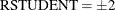are deemed outliers. Observations whose leverage values are greater than the vertical reference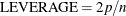, where p is the number of parameters including the intercept and n is the number of observations used, are deemed influential (Rawlings, Pantula, and Dickey 1998). If you specify the LABEL option, then points deemed as outliers or influential are labeled. If you do not specify an ID variable, the observation number within the current BY group is used as the label. If you specify one or more ID variables in one or more ID statements, then the first ID variable you specify is used for the labeling.

RSTUDENTBYPREDICTED <(LABEL)>

plots studentized residuals by predicted values. If you specify the LABEL option, then points deemed as outliers or influential (see the RSTUDENTBYLEVERAGE option for details) are labeled.

SBC <(sbc-options)>

displays Schwarz’s Bayesian information criterion (SBC) for the models examined when you request variable selection with the SELECTION= option in the MODEL statement.

The following sbc-options are available for models where you request the RSQUARE, ADJRSQ, or CP selection method:

LABEL

requests that the model number corresponding to the one displayed in the "Subset Selection Summary" table be used to label the model with the smallest SBC statistic at each value of the number of parameters.

LABELVARS

requests that the list (excluding the intercept) of the regressors in the relevant model be used to label the model with the smallest SBC statistic at each value of the number of parameters.

PRESS

outputs the PRESS statistic to the OUTEST= data set. The values of this statistic are saved in the variable _PRESS_. This option is available for all model selection methods except RSQUARE, ADJRSQ, and CP.

RIDGE=list

requests a ridge regression analysis and specifies the values of the ridge constant k (see the section Computations for Ridge Regression and IPC Analysis). Each value of k produces a set of ridge regression estimates that are placed in the OUTEST= data set. The values of k are saved by the variable _RIDGE_, and the value of the variable _TYPE_ is set to RIDGE to identify the estimates.

Only nonnegative numbers can be specified with the RIDGE= option. Example 97.5 illustrates this option.

If ODS Graphics is enabled (see the section ODS Graphics), then ridge regression plots are automatically produced. These plots consist of panels containing ridge traces for the regressors, with at most eight ridge traces per panel.

If you specify the RIDGE= option, RESTRICT statements are ignored.

RSQUARE

has the same effect as the EDF option.

SIMPLE

displays the sum, mean, variance, standard deviation, and uncorrected sum of squares for each variable used in PROC REG.

SINGULAR=n

tunes the mechanism used to check for singularities. The default value is machine dependent but is approximately 1E–7 on most machines. This option is rarely needed.

Singularity checking is described in the section Computational Methods.

TABLEOUT

outputs the standard errors and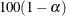% confidence limits for the parameter estimates, the t statistics for testing if the estimates are zero, and the associated p-values to the OUTEST= data set. The _TYPE_ variable values STDERR, LnB, UnB, T, and PVALUE, where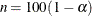, identify these rows in the OUTEST= data set. Thelevel can be set with the ALPHA= option in the PROC REG or MODEL statement. The OUTEST= option must be specified in the PROC REG statement for this option to take effect.

USSCP

displays the uncorrected sums-of-squares and crossproducts matrix for all variables used in the procedure.